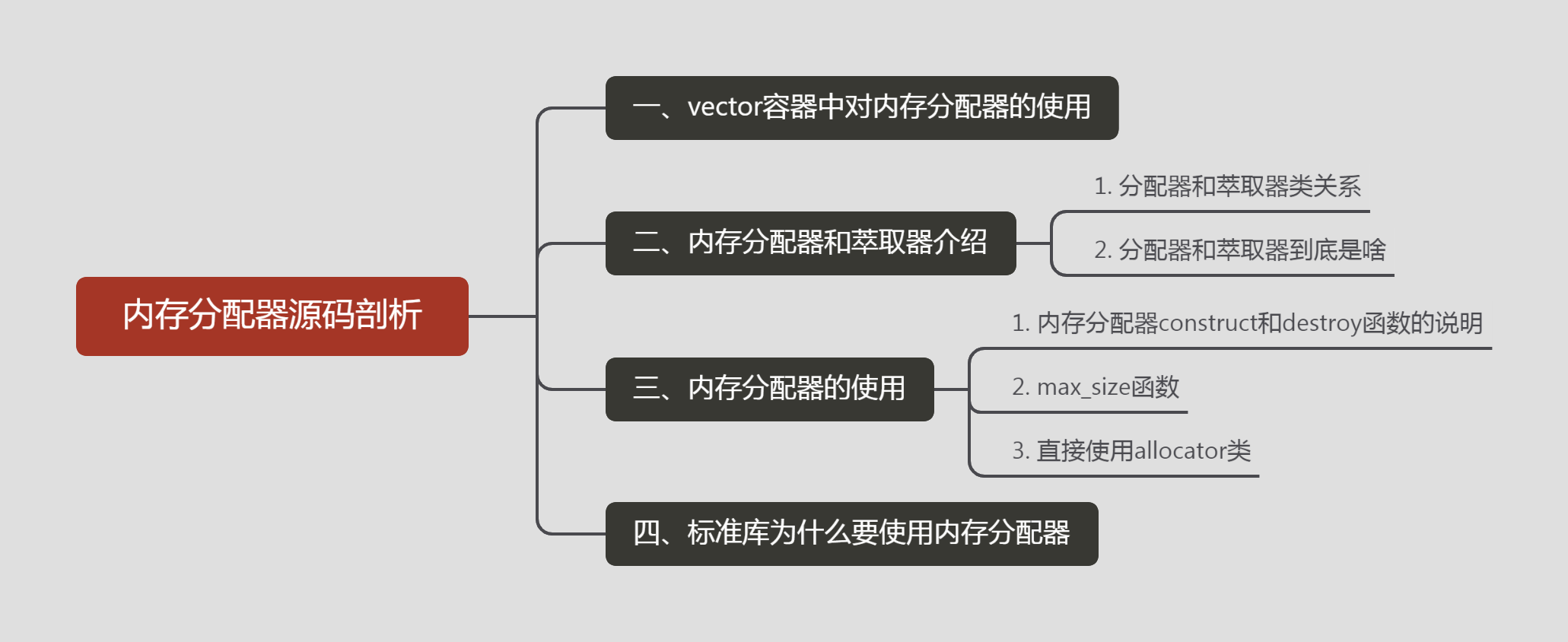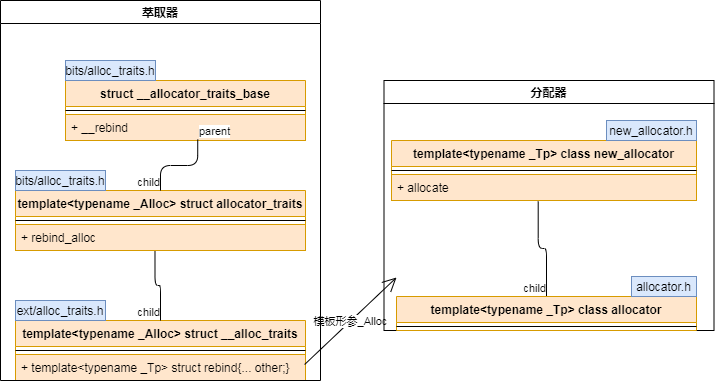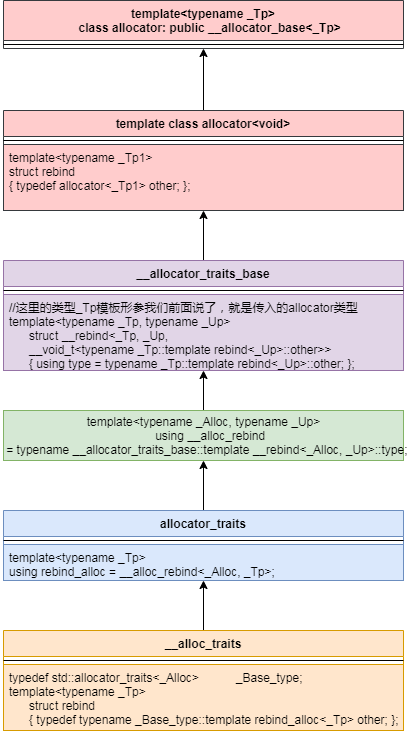#### 一、vector容器中对内存分配器的使用

``````template<typename _Tp, typename _Alloc>
struct _Vector_base
{
typedef typename __gnu_cxx::__alloc_traits<_Alloc>::template
rebind<_Tp>::other _Tp_alloc_type;
typedef typename __gnu_cxx::__alloc_traits<_Tp_alloc_type>::pointer
pointer;

struct _Vector_impl
: public _Tp_alloc_type
{
...
};
...
public:
_Vector_impl _M_impl;

pointer
_M_allocate(size_t __n)
{
typedef __gnu_cxx::__alloc_traits<_Tp_alloc_type> _Tr;
return __n != 0 ? _Tr::allocate(_M_impl, __n) : pointer();
}
...
};
class vector : protected _Vector_base<_Tp, _Alloc>
{...};``````

vector继承于`_Vector_base`，而`_Vector_base`中内存分配又是结构体`_Vector_impl`实现的，这个结构体继承于`_Tp_alloc_type`，类型`_Tp_alloc_type`的完整类型是`__gnu_cxx::__alloc_traits<_Alloc>::template rebind<_Tp>::other`，而根据函数`_M_allocate`最终实现内存分配是通过这样一个方式实现的，如下：

``__gnu_cxx::__alloc_traits<_Tp_alloc_type>::allocate(_Tp_alloc_type, __n);``

#### 二、stl内存分配器和萃取器介绍

##### 1. 分配器和萃取器类关系``````template<typename _Tp>
class allocator: public __allocator_base<_Tp>``````

``````template<typename _Tp>
using __allocator_base = __gnu_cxx::new_allocator<_Tp>;``````

##### 2. 分配器和萃取器到底是啥

``````template<typename _Tp, typename _Alloc>
struct _Vector_base
{
typedef typename __gnu_cxx::__alloc_traits<_Alloc>::template rebind<_Tp>::other _Tp_alloc_type;
struct _Vector_impl: public _Tp_alloc_type
{
...
};
_Vector_impl _M_impl;
//调用函数_M_allocate分配大小为__n的空间
pointer _M_allocate(size_t __n)
{
typedef __gnu_cxx::__alloc_traits<_Tp_alloc_type> _Tr;
return __n != 0 ? _Tr::allocate(_M_impl, __n) : pointer();
}
...
};
template<typename _Tp, typename _Alloc = std::allocator<_Tp> >
class vector : protected _Vector_base<_Tp, _Alloc>;````````````allocator<int> _M_impl;
__gnu_cxx::__alloc_traits<allocator<int>>::allocate(_M_impl, __n);``````

``````static pointer
allocate(_Alloc& __a, size_type __n)
{ return __a.allocate(__n); }``````

``````pointer
allocate(size_type __n, const void* = 0)
{
if (__n > this->max_size())

#if __cpp_aligned_new
if (alignof(_Tp) > __STDCPP_DEFAULT_NEW_ALIGNMENT__)
{
std::align_val_t __al = std::align_val_t(alignof(_Tp));
return static_cast<_Tp*>(::operator new(__n * sizeof(_Tp), __al));
}
#endif
return static_cast<_Tp*>(::operator new(__n * sizeof(_Tp)));
}``````

##### 1. 内存分配器construct和destroy函数的说明

``````//这里入参__p是一个指向当前内存的指针，而入参__val是待存入内存中的值
//这里对new的使用不太好理解，我理解可以转换成：__p = new _Tp(__val);
void construct(pointer __p, const _Tp& __val)
{ ::new((void *)__p) _Tp(__val); }
//销毁就比较好理解一些了，直接调用了元素的析构函数
void destroy(pointer __p) { __p->~_Tp(); }``````

``````#include <iostream>
using namespace std;

int main()
{
int *p = new int;
new (p) int(10);
cout << "*p=" << *p << endl;
}``````

##### 2. max_size函数

``````size_type max_size() const _GLIBCXX_USE_NOEXCEPT
{ return size_t(-1) / sizeof(_Tp); }``````

##### 3. 直接使用allocator类

``````#include <iostream>
#include <bits/allocator.h>
using namespace std;

int main()
{
allocator<int> alloc;
allocator<int>::size_type size = 5;
allocator<int>::pointer ptr = alloc.allocate(size);
for (int i = 0; i< size; i++)
{
alloc.construct(ptr+i, i+1);
}

for (int i = 0; i< size; i++)
{
cout << "alloc[" << i << "]=" << ptr[i] << endl;
}
//这里销毁内存一定要手动调用，因为allocator类的析构函数啥事也没干
alloc.deallocate(ptr, size);

return 0;
}``````

#### 四、标准库为什么要使用内存分配器cpp加油站
Lv1

16

5

1